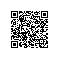# Java8 Lambda表达式.md

（当然这并不是说集合API会常驻永存，毕竟集合API在设计之初并没有考虑到lambda表达式。我们可能会在未来的JDK中添加一个更现代的集合类库）。

# 什么是λ表达式

Lambda 表达式是一个匿名函数，源于数学λ演算。是闭包函数，但闭包并不一定是Lambda 函数。它可以赋值给变量，作为函数参数，作为函数返回值。

### Java 8 lambda表达式的语法

(params) -> expression

(params) -> statement

(params) -> { statements }




Arrays.asList(
"a",
"b",
"d" ).sort( ( e1, e2 ) -> e1.compareTo( e2 ) );

// Java 8之前：

@Override
public void run() {
System.out.println(
"Before Java8, too much code for too little to do"
);}}).start();

//Java 8方式：

"In Java8, Lambda expression rocks !!"
) ).start();

// Java 8之前：

JButton show = new JButton("Show");
@Override
public void actionPerformed(ActionEvent e) {
System.out.println("Event handling without lambda expression is boring");
}});

// Java 8方式：

System.out.println("Light, Camera, Action !! Lambda expressions Rocks");
});



/**
*/
package study.java8.lambda;

import java.util.Arrays;
import java.util.Comparator;
import java.util.List;
import java.util.function.Predicate;

/**
* @author jack 2016年8月18日 下午2:16:53
*/

/**
* @param args
*/
public static void main(String[] args) {

List<Integer> nums = Arrays.asList(1,
23,
4,
56,
7,
8,
10);
// 用stream api
nums.stream()
.sorted()
.forEach(System.out::println);
// lambda表达式本质上也是Collections.sort方法
nums.sort((e1, e2) -> e1.compareTo(e2));
nums.forEach(System.out::println);

// 内部类,Collections.sort方法
nums.sort(new Comparator<Integer>() {

@Override
public int compare(Integer o1, Integer o2) {
return o1.compareTo(o2);
}
});

List<String> languages = Arrays.asList("Java",
"Scala",
"C++",
"Lisp");

System.out.println("Languages which starts with J :");
filter(languages,
(str) -> str.startsWith("J"));

System.out.println("Languages which ends with a ");
filter(languages,
(str) -> str.endsWith("a"));

System.out.println("Print all languages :");
filter(languages,
(str) -> true);

System.out.println("Print no language : ");
filter(languages,
(str) -> false);

System.out.println("Print language whose length greater than 4:");
filter(languages,
(str) -> str.length() > 4);

}

public static void filter(List<String> names, Predicate<String> condition) {
for (String name : names) {
if (condition.test(name)) {
System.out.println(name + " ");
}
}
}

}



Java代码中，对于非常简单的逻辑（比如比较list中element大小），可以使用lambda表达式实现，但是复杂的逻辑千万不要用lamdda表达式，理由只要一个就够了—代码难以维护。理由是:

1. 代码阅读起来不直观
2. 调用栈分析不清楚
3. 后人重构、修改一段匿名函数时很痛苦

## 函数式接口 Comparator

java.util. Comparator


λ表达式本质上是一个匿名方法。让我们来看下面这个例子：

public int add(int x, int y) {return x + y;}


 (int x, int y) -> x + y;


    (x, y) -> x + y; //返回两数之和


    (x, y) -> { return x + y; } //显式指明返回值


    () -> { System.out.println("Hello Lambda!"); }


c -> { return c.size(); }


# λ表达式的类型

λ表达式可以被当做是一个Object（注意措辞）。λ表达式的类型，叫做“目标类型（target type）”。λ表达式的目标类型是“函数接口（functional interface）”，这是Java8新引入的概念。它的定义是：

@FunctionalInterface
public interface Runnable { void run(); }

public interface Callable<V> { V call() throws Exception; }

public interface ActionListener { void actionPerformed(ActionEvent e); }

public interface Comparator<T> { int compare(T o1, T o2);

boolean equals(Object obj); }



Runnable r1 = () -> {System.out.println("Hello Lambda!");};


Object obj = r1;


Object obj = () -> {System.out.println("Hello Lambda!");}; // ERROR! Object is not a functional interface!



 Object o = (Runnable) () -> { System.out.println("hi"); }; // correct


    System.out.println( () -> {} ); //错误! 目标类型不明


    System.out.println( (Runnable)() -> {} ); // 正确


@FunctionalInterface
public interface MyRunnable {public void run();    }



Runnable r1 =    () -> {System.out.println("Hello Lambda!");};

MyRunnable2 r2 = () -> {System.out.println("Hello Lambda!");};


JDK预定义了很多函数接口以避免用户重复定义。最典型的是Function：

@FunctionalInterface
public interface Function<T, R> {   R apply(T t);    }



    @FunctionalInterface    public interface Consumer<T> {        void accept(T t);    }



 @FunctionalInterface    public interface Predicate<T> {
boolean test(T t);
}


# λ表达式的使用

## λ表达式用在何处

Thread oldSchool = new Thread( new Runnable () {
@Override
public void run() {
System.out.println("This is from an anonymous class.");
}} );

System.out.println("This is from an anonymous method (lambda exp).");
} );



## λ表达式与集合类批处理操作（或者叫块操作）**

for(Object o: list) {
// 外部迭代
System.out.println(o);
}



    list.forEach(o -> {System.out.println(o);}); //forEach函数实现内部迭代


Java8为集合类引入了另一个重要概念：流（stream）。一个流通常以一个集合类实例为其数据源，然后在其上定义各种操作。流的API设计使用了管道（pipelines）模式。对流的一次操作会返回另一个流。如同IO的API或者StringBuffer的append方法那样，从而多个不同的操作可以在一个语句里串起来。看下面的例子：

List<Shape> shapes = shapes.stream()
.filter(s -> s.getColor() == BLUE)
.forEach(s -> s.setColor(RED));



filter方法的参数是Predicate类型，forEach方法的参数是Consumer类型，它们都是函数接口，所以可以使用λ表达式。

shapes.parallelStream(); // 或shapes.stream().parallel()




/**

* DistinctPrimary.java

*/

package study.java8.stream;

import java.util.Arrays;

import java.util.List;

import java.util.stream.Collectors;

/**

* @author jack 2016年8月18日 下午1:50:56

*/

public class DistinctPrimary {

/**

* @param args

*/

public static void main(String[] args) {

distinctPrimary(2,

2,

2,

5,

5,

5,

10,

8,

9,

11,

11,

13,

13,

13,

19,

23,

45,

97);

}

// 给出一个Integer类型的数组，找出其中所有不重复的素数

public static void distinctPrimary(Integer... numbers) {

List<Integer> l = Arrays.asList(numbers);

List<Integer> r = l.stream()

.map(e -> new Integer(e))

.distinct()

.filter(e -> Primes.isPrime(e))

.collect(Collectors.toList());

System.out.println("distinctPrimary result is: " + r);

}

}

class Primes {

public static boolean isPrime(int a) {

boolean flag = true;

if (a < 2) {// 素数不小于2

return false;

} else {

for (int i = 2; i <= Math.sqrt(a); i++) {

if (a % i == 0) {// 若能被整除，则说明不是素数，返回false

flag = false;

break;// 跳出循环

}

}

}

return flag;

}

}



    //给出一个String类型的数组，找出其中各个素数，并统计其出现次数    public void primaryOccurrence(String... numbers) {
List<String> l = Arrays.asList(numbers);
Map<Integer, Integer> r = l.stream()
.map(e -> new Integer(e))
.filter(e -> Primes.isPrime(e))
.collect( Collectors.groupingBy(p->p,Collectors.summingInt(p->1)) );

System.out.println("primaryOccurrence result is: " + r);

}



    Collectors.groupingBy(p->p, Collectors.summingInt(p->1))


    //给出一个String类型的数组，求其中所有不重复素数的和    public void distinctPrimarySum(String... numbers) {        List<String> l = Arrays.asList(numbers);        int sum = l.stream()            .map(e -> new Integer(e))            .filter(e -> Primes.isPrime(e))            .distinct()            .reduce(0, (x,y) -> x+y); // equivalent to .sum()        System.out.println("distinctPrimarySum result is: " + sum);    }


reduce方法用来产生单一的一个最终结果。流有很多预定义的reduce操作，如sum()，max()，min()等。

    // 统计年龄在25-35岁的男女人数、比例    public void boysAndGirls(List<Person> persons) {        Map<Integer, Integer> result = persons.parallelStream().filter(p -> p.getAge()>=25 && p.getAge()<=35).            collect(                Collectors.groupingBy(p->p.getSex(), Collectors.summingInt(p->1))        );        System.out.print("boysAndGirls result is " + result);        System.out.println(", ratio (male : female) is " + (float)result.get(Person.MALE)/result.get(Person.FEMALE));    }



## λ表达式的更多用法

 // 嵌套的λ表达式
Callable<Runnable> c1 = () -> () -> { System.out.println("Nested lambda"); };
c1.call().run();

// 用在条件表达式中
Callable<Integer> c2 = true ? (() -> 42) : (() -> 24);    System.out.println(c2.call());

// 定义一个递归函数，注意
protected UnaryOperator<Integer>
factorial = i -> i == 0 ? 1 : i  * this.factorial.apply( i - 1 );
System.out.println(factorial.apply(3));


    int five = ( (x, y) -> x + y ) (2, 3); // ERROR! try to call a lambda in-place


# 其它相关概念

## 捕获（Capture）

Java要求本地变量final或者effectively final的原因是多线程并发问题。内部类、λ表达式都有可能在不同的线程中执行，允许多个线程同时修改一个本地变量不符合Java的设计理念。

## 方法引用（Method reference）

Integer::parseInt //静态方法引用
System.out::print //实例方法引用
Person::new       //构造器引用



    public void distinctPrimarySum(String... numbers) {
List<String> l = Arrays.asList(numbers);
int sum =
l.stream().map(Integer::new)
.filter(Primes::isPrime)
.distinct()
.sum();
System.out.println("distinctPrimarySum result is: " + sum);    }


## 默认方法（Default method）

Java8中，接口声明里可以有方法实现了，叫做默认方法。在此之前，接口里的方法全部是抽象方法。

    public interface MyInterf {
String m1();
default String m2() {
return "Hello default method!";
}
}


    public interface MyInterf {
String m1();
default String m2() {
return "Hello default method!";
}
static String m3() {
return "Hello static method in Interface!";
}
}



## 生成器函数（Generator function）

    Stream.generate(Math::random).limit(5).forEach(System.out::println);使用钉钉扫一扫加入圈子
+ 订阅